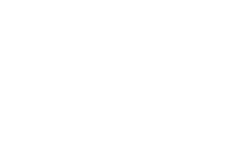# NUMBER BASES

## CONVERSION OF OTHER BASED TO DECIMAL SYSTEM

To convert numbers in other bases to denary system, expand the given number in powers of its base and evaluate.

Examples

1) Convert the following numbers to base ten

(i) 10012 (ii) 255eight (iii) 35416

###(i) 10012 = 1 x 23 + 0 x 22 + 0 x 21 + 1 x 20

= 1 x 8 + 0 x 4 + 0 x 2 + 1 x 1

= 8 + 0 + 0 + 1

= 9ten

(ii) 255eight = 2 x 82 + 5 x 81 + 5 x 80

= 2 x 64 + 5 x 8 + 5 x 1

= 128 + 40 + 5

= 173ten

(iii)   35416 = 3 x 162 + 5 x 161 + 4 x 160

= 3 x 256 + 5 x 16 + 4 x 1

= 768 + 80 + 4

= 852ten

## CHANGING FROM BINARY TO OCTAL AND HEXADECIMAL

To convert from a number system to another one (not denary), it is usual to convert to base ten and then convert the base ten number to the new base number.

However, binary numbers can be converted to octal and hexadecimal numbers because of the fact that 23 = 8 and 22 = 16.

Examples

(1) Convert 110110two to base 8, base 16

(note 23 = 8 and 24 = 16)

(i) 1101102 = (1102) (1102)

= 66eight (1102 = 6ten)

(ii) 1101102 = (00112) (01102)

= 36hex (00112 = 3ten)

2) Convert 1110110two to base 16

1110110 = (01110110)two

= 7616

3) Convert 1000101two to base eight

1000101two = (001)(000)(101)

= 103eight

4) Convert 62eight to base two

62eight = 6                  2

110            010

= 110010two

5)     Change A0316 = A            0           3

1010    0000     0011

= 10100000011two

Also See:

Area

Length

SUBSCRIBE BELOW FOR A GIVEAWAY